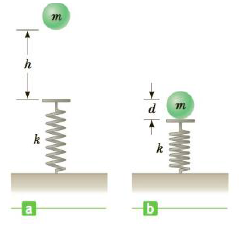Chapter 5, Problem 64AP

Chapter
Section
Textbook Problem

A ball of mass m = 1.80 kg is released from rest at a height h = 65.0 cm above a light vertical spring of force constant k as in Figure P5.64a. The ball strikes the top of the spring and compresses it a distance d = 9.00 cm as in Figure P5.64b.Neglecting any energy losses during the collision, find (a) the speed of the hall just as it touches the spring and (b) the force constant of the spring.(a)

To determine
The speed of the ball when it touches the spring.

Explanation

Given Info:

The mass of the ball is 1.80kg .

The height from which the ball is released is 65.0cm .

The compression of the spring after collision is 9.0cm .

Consider y=0 at the upper end of the spring at initial level.

On the application of conservation of energy,

(KEfKEi)=(PEg,iPEg,f)+(PEs,iPEs,f)

Consider the energy of the ball from the instant it is released to the instant just before it contacts the spring,

12mvf2=0+(mgh0)+(<

(b)

To determine
The force constant of the spring.

Still sussing out bartleby?

Check out a sample textbook solution.

See a sample solution

The Solution to Your Study Problems

Bartleby provides explanations to thousands of textbook problems written by our experts, many with advanced degrees!

Get Started

Find more solutions based on key concepts